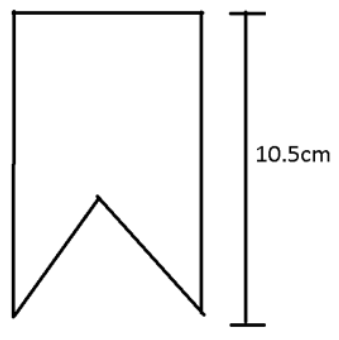Courses
Courses for Kids
Free study material
Offline Centres
MoreLast updated date: 02nd Dec 2023
Total views: 280.8k
Views today: 8.80k

# Anvi is making a book maker like the one shown in figure. How many bookmakers can she make from a 15m long ribbon.Verified
280.8k+ views
Hint: Here in this question we are asked to find the number of bookmarks can be produced by the ribbon. To find the number of bookmarks that can be made by a 15m long ribbon we have to divide the length of the ribbon by the length of the bookmark that she wants to make. Let’s try it.

Complete step-by-step solution:
Given in problem, the length of the ribbon = 15m
From the figure, the length of bookmark = 10.5cm
We already know that , 1m = 100cm
So , the length of the ribbon is $15 \times 100 = 1500cm$
Number of bookmarks can be made by 15m long ribbon is
$= \dfrac{{1500}}{{10.5}} \\ = \dfrac{{1500}}{{105}} \times 10 \\ = \dfrac{{15000}}{{105}} \\ = 142.286$
Therefore, The number of complete bookmarks of 10.5cm that can be prepared from 15m of long ribbon = 142.

Note: In questions like this we do not consider the fraction, when we have to calculate the number of things prepared from a particular long thing because when we are mentioning the number of things prepared we are talking about the things that can be prepared completely but not partially.